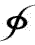See:  The Brunardot Theorem and its Corollaries for the simple, integer  relationships between the below components.
 A = Alpha (motion = y-axis) l = Light
 a = apogee M = Mean (s2 / v)
 c = chord, major o = opposing wave crest
 d = diagonal p = perigee
 E = Energy, Internal r = radius
 f = Force s = soliton
 H = Harmonic T = Tau (time, etc. = x-axis)
 HR = Harmonic Ratio CU = Conceptual Unit
 i = Brunardot Iteration v = vector
 I = Infinity line (x-axis) w = wave crest
 inc. = increment between "i"s x = foci
 K = Unimetric Factor
 To Return to Prior Page "Click" Browser Back Button Until Desired Page Appears